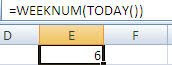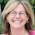## Tuesday, February 2, 2010

### WEEKNUM()

One Date Function that some of you may find useful is =WEEKNUM()
This function returns the week number in the year.
If you want to find out what the current week number is you use the =TODAY() or =NOW() with WEEKNUM.

So, in the example below, =WEEKNUM(TODAY())  returned the value of 6 because today is February 2 2010.Warning: Microsoft points out that the WEEKNUM function is based on the American standard where January 1 is considered the first week of the year even though this year the first week of 2010 only had two days in it. The European standard differs as the first week of the new year has to have at least 4 days in it so this function should not be used if you are presenting or using the data where European standards are used.

1.For completeness sake, here is one way to calculate the ISO weekday from a date in cell A2:

=1+INT((A2-DATE(YEAR(A2+4-WEEKDAY(A2+6)),1,5)+WEEKDAY(DATE(YEAR(A2+4-WEEKDAY(A2+6)),1,3)))/7)

Alternatively, you can use a sligtly shorter array formula:

=INT((A2-SUM(MOD(DATE(YEAR(A2-MOD(A2-2,7)+3),1,2),{1E+99;7})*{1;-1})+5)/7)

And this returns the date of the monday in a specific ISO week (year in E1, weeknumber in E2):

=DATE(\$E\$1,1,1)+(E2-IF(WEEKDAY(DATE(\$E\$1,1,1),2)<5,1,0))*7-WEEKDAY(DATE(\$E\$1,1,1),2)+1

2.Thanks Jan-
For those of you not familiar- The International Standards Organization (ISO) defines an ISO week as always beginning of a Monday.
ISO‘s Week 1 is the first week of the month that has at least 4 days (one of which is Thursday since the week begins on a Monday).